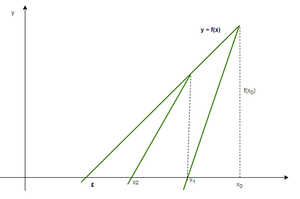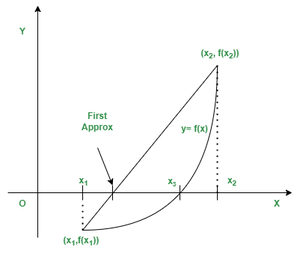# Difference between Newton Raphson Method and Regular Falsi Method

• Last Updated : 31 Dec, 2021

Numerical computations play an important role in solving real-life mathematical problems. Numerical methods are the procedures by applying arithmetic operations one can formulate mathematical problems to find the approximate result. In this, there is no need for algorithms, because numerical methods require the programming logic concept for the implementation. Following is the process of the numerical method,

• Formulated mathematical problems in simple arithmetic operations. This formulation is called the numerical implementation of the problem.
• Programming logic is then developed for numerical implementation. The programming is usually done with some high-level languages like Fortran, Basic, etc.
• The programs are then executed on the computing tools like computers.
• The results are displayed on the screen.

There are different numerical methods for the solutions of the problem but the particular method depends on the situation from which the problem is taken. The following methods come under the concept of obtaining the roots of the equation.

### Newton Raphson Method

The Newton Raphson Method is one of the fastest methods among the bisection and false position methods. In this method, take one initial approximation instead of two. It is the process for the determination of a real root of an equation f(x) = 0 given just one point close to the desired root.Formula for Newton raphson method: x1 = x0 – f(x0)/f'(x0)

Example: Find a root of an equation f(x) = x3 – x – 1

Solution:

Given equation x3 – x – 1 = 0

Using differentiate method the equation is,

∴ f′(x) = 3x2 – 1

Here, f(1) = -1 < 0 and f(2) = 5 > 0

∴ Root lies between 1 and 2

x0 = (1 + 2)/2 = 1.5

• In 1st iteration

f(x0) = f(1.5) = 0.875

f′(x0) = f′(1.5) = 5.75

x1 = x0 – f(x0) / f′(x0)

=1.5 – 0.875/ 5.75

x1 = 1.34783

• In 2nd iteration

f(x1) = f(1.34783) = 0.10068

f′(x1) = f′(1.34783) = 4.44991

x2 = x1 – f(x1)/f′(x1)

= 1.34783 – 0.10068/4.44991

x2 = 1.3252

• In 3rd iteration

f(x2) = f(1.3252) = 0.00206

f′(x2) = f′(1.3252) = 4.26847

x3 = x2 – f(x2)/f′(x2)

=1.3252 – 0.00206/4.26847

x3 = 1.32472

• In 4th iteration

f(x3) = f(1.32472) = 0

f′(x3) = f′(1.32472) = 4.26463

x4 = x3 – f(x3)/f′(x3)

=1.32472 – 0/ 4.26463

x4 = 1.32472

The Approximate root of the equation x3 – x – 1 = 0 using Newton Raphson method is 1.32472.

• It is the best method to solve non-linear equations.
• It is also used to solve non-linear equations, non-linear differentials, and non-linear integral equations.
• The order of convergence is quadratic.
• Easy to implement on a computer.

• This method becomes complicated if the derivative of the function f(x) is not simple.
• This method also fails if f(x) = 0, for some value of x.
• In each iteration, we have to evaluate two quantities f(x) and f'(x) for some x.

### Regular Falsi Method

This method is the same as bisection but it is must faster than the bisection method. This is one of the oldest methods to find the real root of an equation f(x) = 0 and closely resembles with Bisection Method.Graphical Representation of Regula Falsi Method

Formula for regular falsi method:Example: Find a root of an equation f(x) = x3 – x – 1

Solution:

Given equation, x3 – x – 1 = 0

let x = 0, 1, 2

• In 1st iteration

f(1) = -1 < 0 and f(2) = 5 > 0

Root lies between these two points x0 = 1 and x1 = 2

x2 = x0 – f(x0)

= x1 – x0

f(x1) – f(x0)

x2 = 1 – (-1)⋅

= 2 – 1

= 5 – (-1)

x2 = 1.16667

f(x2) = f(1.16667) = -0.5787 < 0

• In 2nd iteration

f(1.16667) = -0.5787 < 0 and f(2) = 5 > 0

Root lies between these two points x0 = 1.16667 and x1 = 2

x3 = x0 – f(x0)

= x1 – x0

f(x1) – f(x0)

x3 = 1.16667 – (-0.5787)

= 2 – 1.16667

= 5 – (-0.5787)

x3 = 1.25311

f(x3) = f(1.25311) = -0.28536 < 0

• In 3rd iteration

f(1.25311) = -0.28536 < 0 and f(2) = 5 > 0

Root lies between these two points x0 = 1.25311 and x1 = 2

x4 = x0 – f(x0)⋅

= x1 – x0

f(x1) – f(x0)

x4 = 1.25311 – (-0.28536)⋅

= 2 – 1.25311

= 5 – (-0.28536)

x4 = 1.29344

f(x4) = f(1.29344) = -0.12954 < 0

• In 4th iteration

f(1.29344) = -0.12954 < 0 and f(2) = 5 > 0

Root lies between these two points x0 = 1.29344 and x1 = 2

x5 = x0 – f(x0)⋅

= x1 – x0

= f(x1) – f(x0)

x5 = 1.29344 – (-0.12954)⋅

= 2 – 1.29344

= 5 – (-0.12954)

x5 = 1.31128

f(x5) = f(1.31128) = -0.05659 < 0

• In 5th iteration

f(1.31128) = -0.05659 < 0 and f(2) = 5 > 0

Root lies between these two points x0 = 1.31128 and x1 = 2

x6 = x0 – f(x0)⋅

= x1 – x0

= f(x1) – f(x0)

x6 = 1.31128 – (-0.05659)⋅

= 2 – 1.31128

= 5 – (-0.05659)

x6 = 1.31899

f(x6) = f(1.31899) = -0.0243 < 0

• In 6th iteration

f(1.31899) = -0.0243 < 0 and f(2) = 5 > 0

Root lies between these two points x0 = 1.31899 and x1 = 2

x7 = x0 – f(x0)⋅

= x1 – x0

f(x1) – f(x0)

x7 = 1.31899 – (-0.0243)⋅

= 2 – 1.31899

= 5 – (-0.0243)

x7 = 1.32228

f(x7) = f(1.32228) = -0.01036 < 0

• In 7th iteration

f(1.32228) = -0.01036 < 0 and f(2) = 5 > 0

Root lies between these two points x0 = 1.32228 and x1 = 2

x8 = x0 – f(x0)⋅

x1 – x0

f(x1) – f(x0)

x8 = 1.32228 – (-0.01036)⋅

= 2 – 1.32228

= 5 – (-0.01036)

x8 = 1.32368

The approximate root of the equation x3 – x – 1 = 0 using the Regula Falsi method is 1.32368.

• Its convergence is faster than the Bisection Method.
• It is easy to implement on a computer.
• Before starting the next iteration; only need to find id f(xn + 1)